## Example Questions

### Example Question #116 : Trigonometry

What is the domain of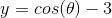?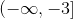Does not exist.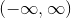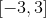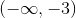Explanation:

The domain of a function is referring to the x values that can be plugged into the function and produce a value.

The domain of the parent function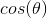has a domain from negative infinity to positive infinity.

Theterm only shifts the function down three units, which will not affect the domain of the cosine graph.

Therefore, the answer is.

### Example Question #117 : Trigonometry

Given a function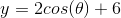, what is a valid domain?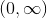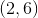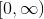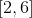Explanation:

The functionis related to the parent function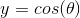.

The domain of the parent function is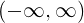.  The valuesandwill not affect the domain of the curve.

The answer is.

### Example Question #118 : Trigonometry

What is the domain of the following trigonometric equation: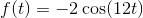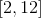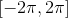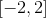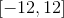Explanation:

For sine and cosine, they can take any for, thus the domain is all real numbers or:### Example Question #119 : Trigonometry

What is the domain of the function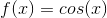?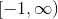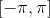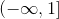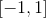Explanation:

The domain of a function refers to all possible values offor which an answer can be obtained. Cosine, as a function, cycles endlessly betweenand(subject to modifiers of the amplitude). Because there is no real number value that can be inserted intoin this case which does not produce a value betweenand, the domain of cosine is effectively infinite.

### Example Question #120 : Trigonometry

What is the domain of the function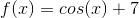?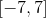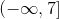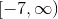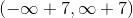Explanation:

The domain of a function refers to all possible values offor which an answer can be obtained. Cosine, as a function, cycles endlessly betweenand(subject to modifiers of the amplitude). Because there is no real number value that can be inserted intoin this case which does not produce a value betweenand, the domain of cosine is effectively infinite.

Note that addingto the end of the equation changes nothing with respect to domain, as there is no such thing as "infinity plus seven", nor "negative infinity plus seven". The function is still infinite in domain even when shifted upunits.

### Example Question #121 : Trigonometry

What is the domain of the function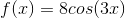?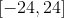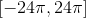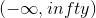The domain of a function refers to all possible values offor which an answer can be obtained. Cosine, as a function, cycles endlessly betweenand(subject to modifiers of the amplitude). Because there is no real number value that can be inserted intoin this case which does not produce a value betweenand, the domain of cosine is effectively infinite.
Note that in this case, neither thenor theon the outside affect the domain of the function. They do affect the amplitude, which means the value for range will change, but there is no such thing as "three times infinity" nor "three times negative infinity", so the effective domain remains infinite.